Search

# Artificial Intelligence, poker and regret. Part 1

## An introduction to Counterfactual Regret Minimisation and poker.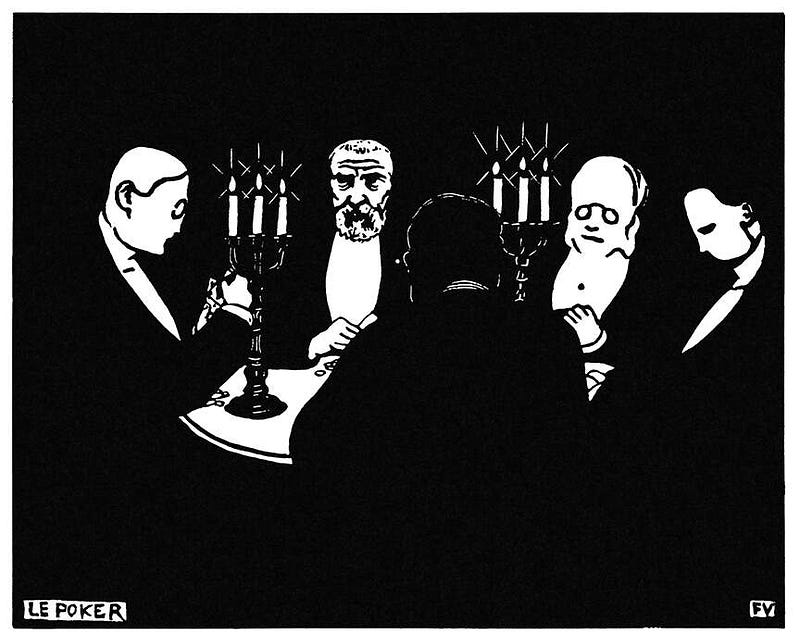We’ll be putting out a 3 part blog series giving an introduction to Counterfactual Regret Minimisation (CFR), which is a reinforcement learning algorithm that has recently beaten a number of professional poker players. We’ll be starting with an introduction to the simpler version the algorithm, Regret Matching, (with code) then in the later parts of the series, share some of the findings from our own research and finally share an example of the CFR algorithm that plays a version of poker.

Counterfactual regret algorithm is a self playing A.I model. Essentially two AI agents playing against each other and learning the game from scratch. In fact in many cases, it’s an agent playing against itself, so it learns twice as fast (it’s important to note that although it does play itself, it is in no way smart enough to actually understand it’s own last move from the Opponent’s position.)

Unlike many recent important breakthroughs in A.I Research, like Deepmind’s AlphaGo, CFR does not rely on Neural Networks to calculate probabilities or the value of a particular move. Instead by playing itself over millions if not billions of games, it can begin to sum up the total amount of regret for each action it has taken in particular positions.

What’s exciting about this algorithm is that as it plays, it gets closer and closer to an optimal strategy for the game. Namely toward a Nash Equilibrium. It has proven itself across a number of games and domains, most interestingly that of Poker, specifically no-limit Texas Hold ’Em. At this point in time it’s the best Poker AI algorithm we have.

### Regret Matching.

Regret matching (RM) is an algorithm that seeks to minimise regret about its decisions at each step/move of a game. As the name suggests, it learns from past behaviours to inform future decisions by favouring the action it regretted not having taken previously.

In this model, there is both positive regret and negative regret. Where negative regret is defined as you would expect; the regret of having taken a particular action in a particular situation. It means the agent would have done better had it not chosen this action in this situation.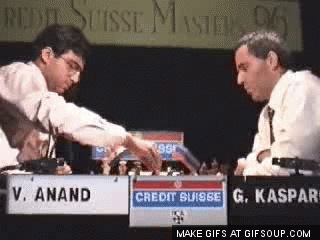Positive regret is also as you would expect; it is a mechanism by which the agent tracks actions that resulted in a positive outcome. (I personally think it should be called something else, but whatever.)

After each game that the Agent plays against itself, the negative and positive regrets that it just experienced in the latest game are added to the summed totals across all other games it has played and it’s new strategy is computed.

To put it in simple terms, via probabilities, it favours actions that in the past have resulted in positive outcomes and avoids actions that have resulted in negative ones.

Alongside other learning mechanisms, human beings learn through regret — we will attempt something and if it fails and incurs a negative emotional response, we’ll track this regret and avoid the attempt next time. Using the game of ‘Scissors-Paper-Rock(SPR)’ as an example, if we presented Rock when the opponent’s gesture is Paper, we regret not having chosen Scissor.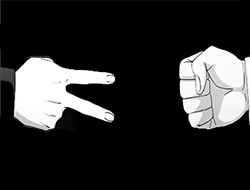This model moves toward a Nash Equilibrium in a Zero-Sum Game, which for those not versed in game theory is just game where each agent’s win or loss is exactly balanced by the loss or win of the other agent. For example, Scissors, Paper, Rock, is a Zero-Sum Game. When you beat your opponent, you win 1, they lose -1, which totals Zero.

In the following section, we formalise SPR as a mathematical problem, then we explain the Regret Matching while walking through a Python program that finds Nash Equilibrium strategy in SPR by RM. Regret Matching is not the holistic algorithm currently beating Professional Poker Players, but it is the foundation of that Algorithm.

### Scissors-Paper-Rock

Here we formalise the Scissors-Paper-Rock (SPR) as a normal-form, zero-sum and two-player game in which Nash Equilibrium exists. While this sentence contains a lot of information, let’s break it down into components. First, SPR can be defined as tuple < N, A, u >, formally known as normal form:

• N = 1,….n is a finite set of n players. In SPR, normally ​N = {1,2}

• Si is a finite set of actions. Here each player has same action choices , so S = { S , P , R }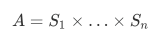is the all possible combinations of simultaneous actions of all players. For example, A = {(R,R),…….,(S,P)}​, and each combination is called an action profile.

• U is a function mapping each action profile to a vector to utilities/payoffs for each player. For example, (R,P) = (-1,1)​ means the reward to player1 is -1 if he is beaten by player 2 by presenting Rock to opponent’s Paper. Hence we can define a utility matrix for player 1 as following, assuming the row player is player 1: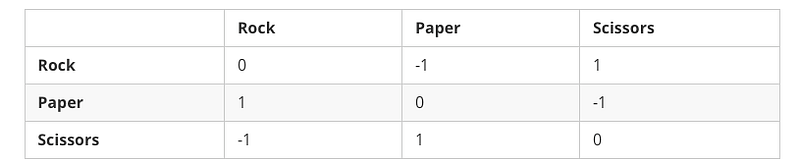Besides, we also define strategy σᵢ(s)​ as the probability of player ᵢ​ chooses action ​sS. When a player uses regret-matching to update his strategy, σᵢ(s)​​ is proportional to the cumulative regret of s​. Notice that each utility vector sums to 0, for which reason we call it a zero-sum game. In any two-player zero-sum game, when both players adhere to regret-matching, their averaged strategy converges to a Nash Equilibrium, i.e, both are able to maximise own expected utility: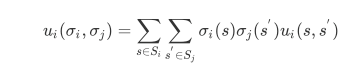But enough math abstractions for now. Without further ado, let’s gain some concrete ideas of how Regret Matching actually works in practice. In this tutorial, we will write a simple program that implements the RM algorithm to play SPR game. We assume the reader has basic knowledge of Python programming language and preferably a touch of Numpy library.

We begin with importing the modules needed in this program.

Then we define primitives of Scissors-Paper-Rock game. The ‘utilities’ is the aforementioned utility function that determines the utility value for an action profile. As a convention, we define the row player is player 1 and the column player is player 2. So to query the utility value for player 1 given the action profile (s1 = Rock, s2= Paper), we call utilities.loc['ROCK', 'PAPER'].

Each player is responsible for maintaining his own strategy and regret statistics. For the time being, we only present a class skeleton but we will come back to go through implementation details later. There are a few variables worth explaining:

• Strategy: correspond to σ(s), strategy vector, representing the current probability of taking action s​.

• Strategy Sum: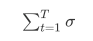is the sum of strategy vectors since the beginning of game up to now.

• Regret_sum: is the sum of regret vectors at each iteration.

• avg_strategy: normalised strategy sum

Definition of game/environment. We have two players, Alasdair and Calum, playing Scissors-Paper-Rock for 1000 rounds unless the max_game is explicitly specified. The ‘play_regret_matching’ function sufficiently describes the process of original regret matching:

1. Compute a regret-matching strategy profile from cumulative regrets.

2. Select each player action profile according the strategy profile

3. Compute player regrets and add them to player cumulative regrets.

Now let’s implement the unfinished player class, starting with the ‘regret’ and ‘action’ function. Considering an action profile ​(Rock, Paper), the corresponding regret of not having taking an action is essentially the utility difference between the action and opponent action, i.e,(Rock = -1, Scissor =1, Paper = 0)​, Scissor is definitely missed.

The ‘update_strategy’ function coincides with the regret matching algorithm’s core idea: “selects actions in proportion to positive regrets of not having chosen them in the past”, thus strategy is in fact the normalisation of positive regret sum. Note however, when there is no positive regret (i.e, the last play was perfect), we adopt to a random policy to minimise exposing the bias towards an action as such bias can be exploited by the opponent,

Now we can start the party! Try to run it multiple times, you may observe that simple regret matching does not always produce uniformly distributed winner counts, whereas averaged regret matching does. If you print the ‘num_wins’ at each intermediate iteration in simple regret matching, you might even notice the winner is periodically between p1 and p2. This is due to the exploitability of simple regret matching. Imagining you are aware that the opponent prefers Scissors, you may then favour Rocks in the next few round, and it takes a while for opponent to regret his bias.

However, averaging leads to a perfect strategy in SPR — play randomly. This is the best response to whatever fixed strategy the opponent chooses, and leaves no exploitable information in each action selection.

Full source code is available at : https://gist.github.com/namoshizun/7a3b820b013f8e367e84c70b45af7c34

The next blog in this series will be on the use of CFR in Auctions, in particular in Paid Search Auctions.

By Di Lu, Alasdair Hamilton and Calum Hamilton

remi.ai

Stay tuned on the Remi AI blog as we build out the complete supply chain offering!

Or, if you're ready to start seeing the benefits of A.I-powered inventory management, start the journey here.

Who are we?

Remi AI is an Artificial Intelligence Research Firm with offices in Sydney and San Francisco. We have delivered inventory and supply chain projects across FMCG, automotive, industrial and corporate supply and more.

Want to know more? Sign up to our newsletter for the latest information from us and other knowledgeable folk in the market.

See All